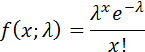SORTIE-ND
Software for spatially-explicit simulation of forest dynamics

# Math and functional forms

## Randomness in SORTIE-ND

SORTIE uses the Mersenne Twister algorithm to generate random numbers. You can supply a seed to the random number generator in the Plot parameters. Supplying a seed with a value other than 0 causes the random number generator to produce the same sequence of values each time, which would cause two runs of the same parameter file to come out identically. Setting the seed value to zero causes SORTIE to choose its own random seed based on the current machine time.

## Probability distribution functions

An important part of SORTIE's functionality is simulating stochasticity in natural processes. In order to do this, many behaviors use a random draw on a probability distribution function to make decisions.

The normal distribution is:where σ is the function standard deviation. Mean is zero.

The lognormal distribution is:where λ is the function mean and σ is the standard deviation.

The Poisson distribution is:where λ is the function mean.

The negative binomial distribution is:where u is the function mean and k is the clumping parameter. This is Equation 3.103 from Hilborn and Mangel.

The gamma distribution is:where a is the shape parameter and s is the scale parameter. The function mean is a*s.